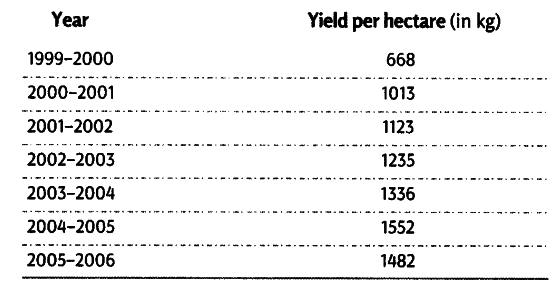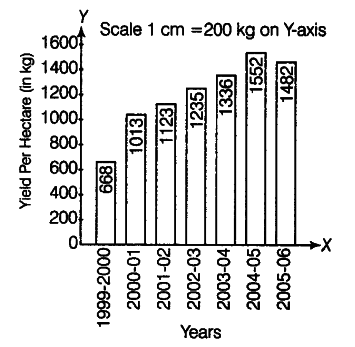# Rice yield per hectare is given below

Rice yield per hectare is given below. Show the data diagrammatically.The above data can be presented with the help of a simple bar diagram, since only one variable is to be presented. The figure is drawn below